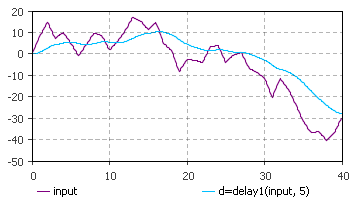AnyLogic

# delay1

delay1 is the system dynamics function that returns an exponential delay of the input.

The plot below illustrates how the function works:This function can be called in formulas of system dynamics variables and has two notations:

• delay1(input, delayTime, initialValue)

input can be a flow variable, or a numeric expression of any complexity.

delayTime can be either a constant or a numeric expression (e.g. a function call, or a numeric parameter). It should return a positive number: delay1 function with zero or negative delay time returns NaN (not a number).

• delay1(input, delayTime)

A simplified notation of the function. It is used when the initialValue is zero.

#### Units

input — unit

delayTime — time

initialValue — unit

delay1() — unit

The output units are the same as the input ones.